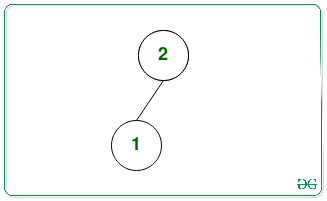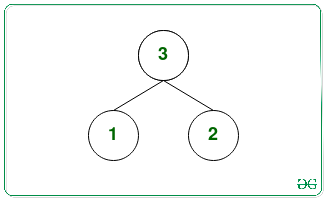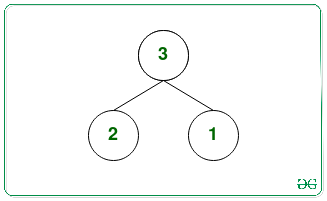GFG App
Open AppBrowser
Continue

# Find min and max values among all maximum leaf nodes from all possible Binary Max Heap

Given a positive integer N, the task is to find the largest and smallest elements, from the maximum leaf nodes of every possible binary max-heap formed by taking the first N natural numbers as the nodes’ value of the binary max-heap.

Examples:

Input: N = 2
Output: 1 1
Explanation:
There is only one maximum binary heap with the nodes {1, 2}:In the above tree, maximum leaf node value = 1.
Therefore, the largest element is 1 and the smallest element is also 1.

Input: N = 3
Output: 2 2
Explanation:
There are two possible maximum binary heaps with the nodes {1, 2, 3}:The maximum leaf nodes of first and second heaps are 2 and 2 respectively.
Therefore, the largest element is 2 and the smallest element is also 2.

Naive Approach: The simplest approach is to generate all possible max binary heaps that can be formed from first N natural numbers and keep track of the smallest and largest leaf node values among all the maximum leaf nodes in all the heaps.

Time Complexity: O(N*N!)
Auxiliary Space: O(1)

Efficient Approach: The above approach can be optimized based on the following observations:

1. The max heap is a complete binary tree, therefore, the height and count of the number of leaf nodes are fixed.
2. In the max heap, the value of the node is always greater than or equal to the children of that node.
3. The maximum leaf node value is always greater than or equal to the number of leaves in the tree. Therefore, the maximum value of a leaf node will be minimized if the smallest numbers are placed at the leaf nodes. And will be equal to the number of leaves node.
4. One more property of the max heaps is as one will go down the tree the values of nodes decrease. Therefore, the maximum value of a node will be maximized if a number is placed at the leaf node with the least possible depth. So if D is the depth of that node then the maximum possible value of the node will be equal to the N-D.
5. If D is the depth of the max Heap then the possible Depths of the leaf nodes are D and D-1 only, as the heaps are the complete binary tree.
6. If V is a leaf node then (2*V) must be greater than N. Therefore, the count of leaf nodes is equal to the, (N – N/2).

Follow the steps below to solve the problem:

`numberOfleaves = (N- N/2).`
`D = ceil(log2(N+1))-1`
• If N+1 is not a power of 2 and D is greater than 1, then there must exit a leaf node at depth D-1. Therefore, then decrement D by 1.
• Finally, after completing the above steps, print the largest value as (N-D) and the smallest value as numberOfleaves.

Below is the implementation of the above approach:

## C++

 `// C++ program for the above approach` `#include ` `using` `namespace` `std;`   `// Function to find largest and smallest` `// elements from the maximum leaf nodes` `// values from all possible binary max heap` `void` `leafNodeValues(``int` `N)` `{` `    ``// Stores count of leaf nodes` `    ``int` `numberOfLeaves = (N - N / 2);`   `    ``// Stores minDepth with N` `    ``int` `minDepth = ``ceil``(log2(N + 1)) - 1;`   `    ``// Increment N by 1` `    ``N++;` `    ``// If N is not power of 2 and minDepth` `    ``// is greater than 1` `    ``if` `(minDepth > 1 && (N & (N - 1)))` `        ``minDepth--;`   `    ``// Print the smallest and largest possible` `    ``// value of a leaf node` `    ``cout << numberOfLeaves << ``' '` `         ``<< N - minDepth - 1;` `}`   `// Driver Code` `int` `main()` `{` `    ``// Given Input` `    ``int` `N = 2;`   `    ``// Function Call` `    ``leafNodeValues(N);`   `    ``return` `0;` `}`

## Java

 `// Java program for the above approach` `class` `GFG{` `    `  `// Function to find largest and smallest` `// elements from the maximum leaf nodes` `// values from all possible binary max heap` `static` `void` `leafNodeValues(``int` `N)` `{` `    `  `    ``// Stores count of leaf nodes` `    ``int` `numberOfLeaves = (N - N / ``2``);` ` `  `    ``// Stores minDepth with N` `    ``int` `minDepth = (``int``)Math.ceil(Math.log(N + ``1``) /` `                                  ``Math.log(``2``)) - ``1``;` ` `  `    ``// Increment N by 1` `    ``N++;` `    `  `    ``// If N is not power of 2 and minDepth` `    ``// is greater than 1` `    ``if` `(minDepth > ``1` `&& (N & (N - ``1``)) != ``0``)` `        ``minDepth--;` ` `  `    ``// Print the smallest and largest possible` `    ``// value of a leaf node` `    ``System.out.println(numberOfLeaves + ``" "` `+ ` `                      ``(N - minDepth - ``1``));` `}`   `// Driver code` `public` `static` `void` `main(String[] args)` `{` `    `  `    ``// Given Input` `    ``int` `N = ``2``;` ` `  `    ``// Function Call` `    ``leafNodeValues(N);` `}` `}`   `// This code is contributed by divyesh072019`

## Python3

 `# Python 3 program for the above approach` `from` `math ``import` `ceil,log2`   `# Function to find largest and smallest` `# elements from the maximum leaf nodes` `# values from all possible binary max heap` `def` `leafNodeValues(N):` `  `  `    ``# Stores count of leaf nodes` `    ``numberOfLeaves ``=` `(N ``-` `N ``/``/` `2``)`   `    ``# Stores minDepth with N` `    ``minDepth ``=` `ceil(log2(N ``+` `1``)) ``-` `1``;`   `    ``# Increment N by 1` `    ``N ``+``=` `1` `    `  `    ``# If N is not power of 2 and minDepth` `    ``# is greater than 1` `    ``if` `(minDepth > ``1` `and` `(N & (N ``-` `1``))):` `        ``minDepth ``-``=` `1`   `    ``# Print the smallest and largest possible` `    ``# value of a leaf node` `    ``print``(numberOfLeaves, N ``-` `minDepth ``-` `1``)`   `# Driver Code` `if` `__name__ ``=``=` `'__main__'``:` `    ``# Given Input` `    ``N ``=` `2` `    `  `    ``# Function Call` `    ``leafNodeValues(N)` `    `  `    ``# This code is contributed by SURENDRA_GANGWAR.`

## C#

 `// C# program for the above approach` `using` `System;` `class` `GFG {` `    `  `    ``// Function to find largest and smallest` `    ``// elements from the maximum leaf nodes` `    ``// values from all possible binary max heap` `    ``static` `void` `leafNodeValues(``int` `N)` `    ``{` `         `  `        ``// Stores count of leaf nodes` `        ``int` `numberOfLeaves = (N - N / 2);` `      `  `        ``// Stores minDepth with N` `        ``int` `minDepth = (``int``)Math.Ceiling(Math.Log(N + 1) /` `                                      ``Math.Log(2)) - 1;` `      `  `        ``// Increment N by 1` `        ``N++;` `         `  `        ``// If N is not power of 2 and minDepth` `        ``// is greater than 1` `        ``if` `(minDepth > 1 && (N & (N - 1)) != 0)` `            ``minDepth--;` `      `  `        ``// Print the smallest and largest possible` `        ``// value of a leaf node` `        ``Console.WriteLine(numberOfLeaves + ``" "` `+` `                          ``(N - minDepth - 1));` `    ``}`   `  ``static` `void` `Main() ` `  ``{` `    `  `    ``// Given Input` `    ``int` `N = 2;` `  `  `    ``// Function Call` `    ``leafNodeValues(N);` `  ``}` `}`   `// This code is contributed by decode2207.`

## Javascript

 ``

Output:

`1 1`

Time Complexity: O(log(N))
Auxiliary Space: O(1)

My Personal Notes arrow_drop_up